Home > CCA2 > Chapter 12 > Lesson 12.1.3 > Problem12-43

12-43.
1. Solve each equation for 0° ≤ θ < 360°, or if you prefer, 0 ≤ θ < 2π. You may need your calculator, but remember that it only gives one answer. Homework Help ✎

1. sin(θ) = 0.5

2. cos(θ) = −0.5

3. 4 tan (θ) − 4 = 0

4. 3 sin2(θ) = 1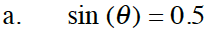Use inverse sine on your calculator to find one solution.
sin−1(0.5) = θ

It should tell you the answer is 30°.

Draw a unit circle to find the other angle that makes sin(θ) = 0.5. The sine function is positive in quadrants I and II, so draw a reference angle of 30° in each quadrant.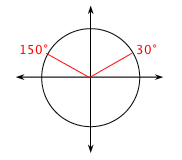Follow the steps in part (a).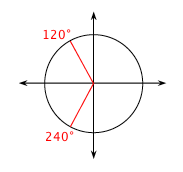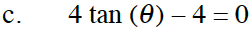First solve the equation for tan(θ).
tan(θ) = 1.

Follow the steps in part (a).

45° and 225°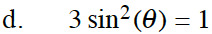First solve the equation for sin(θ).

$\sin(\theta) \ = \pm \sqrt{\frac{1}{3}}$

Follow the steps in part (a). There are 4 solutions.